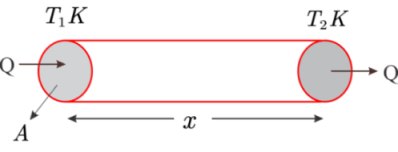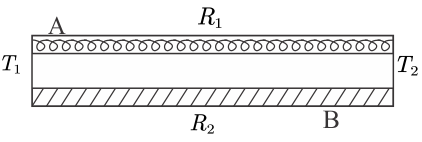# Thermal resistance and thermal conductance (series & parallel)

## Thermal Resistance

We already know about rate of heat flow $H$, is also known as heat current We will now define Thermal Resistance.

The opposition of a body to the flow of heat through it is called thermal resistance of the body.
Thermal resistance is analogues to electric resistance which opposes flow of electric current in a conductor. Greater is the thermal conductivity of a material, the smaller is its thermal resistance.
Let $x$ be the length and $A$ be the area of cross-section of a bar. $T_1$ and $T_2$ are the temperatures of the hot and cold ends of the bar.Figure for finding thermal resistance in a bar of length $x$ and cross-sectional area $A$. In the steady state rate of flow of heat is given by
$$\bbox[aqua,5px,border:2px solid red]{ H = \frac{Q}{t} = \frac{{KA}({T_1}-{T_2})}{x} }$$ or, $$\frac{T_1 T_2}{H} = \frac{x}{KA} \tag{1}$$ Now from ohm's law, electric resistance is given by
$$R =\frac{V}{I}$$ This equation shows that electric resistance is the ratio of potential difference and electric current. Similarly, the ratio of the temperature difference between ends of the conductor to the heat current through it is called thermal resistance. So, from equation (1) \begin{equation*} R_H =\frac{T_1-T_2}{H}-\frac{\Delta T}{H}\\ \bbox[aqua,5px,border:2px solid red]{ \Rightarrow R_H=\frac{\Delta T}{H}=\frac{x}{KA}} \\ \color{blue}{\text{equation for thermal resistance offered to the heat current}} \end{equation*}

### Unit and dimensions of thermal resistance $R_H$

As, $$R_H=\frac{\Delta T}{\Delta H}$$ it’s SI unit is $$\frac{K}{Js^{-1}}=\frac{K}{W}=KW^{-1}$$ it's dimensions are, \begin{align*} Dimensions&=\frac{\left[ K \right]}{\left[ ML^2T^{-2} \right] -\left[ T^{-1} \right]}\\ &=\left[ M^{-1}L^{-2}T^3K \right]\\ \end{align*} Good heat conductors has low thermal resistance and a bad conductor of heat has high thermal resistance.

## Thermal conductance:-

Thermal conductance is the reciprocal of thermal resistance. So,
Thermal conductance, \begin{align*} G &= \frac{1}{R}\\ &= \frac{H}{\Delta T}= \frac{{KA}}{x} \end{align*}

### Thermal conductance for Series connection of rods

Consider two rods of thermal resistance $R_1$ and $R_2$as shown in the figure. Two free ends of the rod one kept at temperatures $T_1$ and $T_2$ where $T_1 > T_2$ as shown below in the figure.Figure for finding Thermal conductance for Series connection of rods. When the steady state is reached there is no absorption of heat in any part of the rod. So, any heat that goes through the first rod also goes through the second rod. Due to this same heat current passes through the two rods. Such a connection of rods is called series connection. Let $T$ be the temperature of the junction of two rods. Now heat current through first rod is \begin{align*} & H=\frac{T_1-T}{R_1} \\ & or, \\ & T_1-T=R_1H \tag{2} \end{align*} And that through second rod is \begin{align*} & H=\frac{T-T_2}{R_2} \\ & or, \\ & T-T_2=R_2H \tag{3} \end{align*} Adding equations (2) and (3) we get \begin{align*} & T_1-T_2=(R_1+R_2)H \\ & or, \\ & H=\frac{T_1-T_2}{R_1+R_2} \\ \end{align*} Thus two rods together is equivalent to a single rod of thermal resistance $R_1+R_2$ If we join more than two rods in series, the equivalent thermal resistance is given by $$\bbox[aqua,5px,border:2px solid red]{ R = R_1 +R_2 +R_3 .....}$$

### Thermal conductance for parallel connection of rods

Again consider the figure given belowFigure for finding Thermal conductance for Parallel connection of rods. Where two rods are joined at their ends.
From the figure me can say that left ends of both rods A and B are kept at temperature and the right ends one kept at temperature $T_2$. So, there is same temperature difference maintained at the ends of each rod.
Such a connection of rods is called a parallel connection.
The heat current going through the first rod is $$H_1=\frac{T_1 - T_2}{R_1}$$ and that through the second rod is, $$H_2=\frac{T_1-T_2}{R_2}$$ Total heat current going through left end is \begin{align*} H&=H_1+H_2 \\ & =\left( T_1-T_2 \right)\left( \frac{1}{R_1}+\frac{1}{R_2} \right) \end{align*} or, \begin{align*} H=\frac{T_1-T_2}{R} \end{align*} where, \begin{align*} \frac{1}{R}=\frac{1}{R_1}+\frac{1}{R_2} \end{align*} thus, the system of two rods is equivalent to a single rod of thermal resistance R given by above equation.
If more than two rods are joined in parallel, the equivalent thermal resistance is given by \begin{equation*} \bbox[aqua,5px,border:2px solid red]{ \frac{1}{R} = \frac{1}{R_1} + \frac{1}{R_2} + \frac{1}{R_3}+.... } \end{equation*}